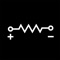## Ohm's Law calculators for current, voltage, resistance, and power# Ohm Law Calculator

by NitrioOhm's Law calculators for current, voltage, resistance, and power. You may key in two of the four factors in Ohm's Law. They are Power (P) or (W), measured in Watts, Voltage (V) or (E), measured in Volts, Current or Amperage (I), measured in Amps (Amperes), and Resistance (R) measured in Ohms.### App Details

Version
1.7
Rating
(1)
Size
14Mb
Genre
Utilities Productivity
Last updated
July 9, 2020
Release date
March 4, 2012

### App Screenshots### App Store Description

Ohm's Law calculators for current, voltage, resistance, and power. You may key in two of the four factors in Ohm's Law. They are Power (P) or (W), measured in Watts, Voltage (V) or (E), measured in Volts, Current or Amperage (I), measured in Amps (Amperes), and Resistance (R) measured in Ohms.

The needed factor will be calculated for you when you key in the needed value.

The various possible formulas for Ohm's law are as follows:
P = power in watts
E = voltage in volts
I = current in amps
R = resistance in ohms

P = E2 / R
P = I2 * R
P = E * I

E = sqrt(P * R)
E = P / I
E = I * R

I = E / R
I = P / E
I = sqrt(P / R)

R = E / I
R = E2 / P
R = P / I2

Thanks for your support and do visit nitrio.com for more apps for your iOS devices.

Disclaimer:
AppAdvice does not own this application and only provides images and links contained in the iTunes Search API, to help our users find the best apps to download. If you are the developer of this app and would like your information removed, please send a request to [email protected] and your information will be removed.